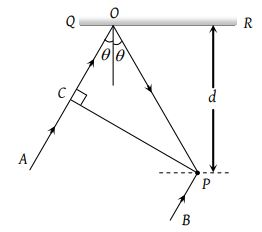Unpolarized light falls on two polarizing sheets placed one on top of the other. What must be the angle between the characteristic directions of the sheets if the intensity of the final transmitted light is one-third the maximum intensity of the first transmitted beam?

(1) 75°

(2) 55°

(3) 35°

(4) 15°

Concept Questions :-

Polarization of light
High Yielding Test Series + Question Bank - NEET 2020

Difficulty Level:

Unpolarized light of intensity 32Wm–2 passes through three polarizers such that transmission axes of the first and second polarizer makes and angle 30° with each other and the transmission axis of the last polarizer is crossed with that of the first. The intensity of final emerging light will be

(1) 32 Wm–2

(2) 3 Wm–2

(3) 8 Wm–2

(4) 4 Wm–2

Concept Questions :-

Polarization of light
High Yielding Test Series + Question Bank - NEET 2020

Difficulty Level:

In the visible region of the spectrum the rotation of the place of polarization is given by $\theta =a+\frac{b}{{\lambda }^{2}}$. The optical rotation produced by a particular material is found to be 30° per mm at $\lambda =5000$Å and 50° per mm at $\lambda =4000\text{Å}$. The value of constant a will be

(1) $+\frac{50°}{9}$per mm

(2) $-\frac{50°}{9}$per mm

(3) $+\frac{9°}{50}$per mm

(4) $-\frac{9°}{50}$per mm

Concept Questions :-

Polarization of light
High Yielding Test Series + Question Bank - NEET 2020

Difficulty Level:

When an unpolarized light of intensity I0 is incident on a polarizing sheet, the intensity of the light which does not get transmitted is

(1) Zero

(2) I0

(3) $\frac{1}{2}{I}_{0}$

(3) $\frac{1}{4}{I}_{0}$

Concept Questions :-

Polarization of light
High Yielding Test Series + Question Bank - NEET 2020

Difficulty Level:

When the angle of incidence on a material is 60°, the reflected light is completely polarized. The velocity of the refracted ray inside the material is (in ms–1)

1. 3 × 108

2. $\left(\frac{3}{\sqrt{2}}\right)×{10}^{8}$

3. $\sqrt{3}×{10}^{8}$

4. 0.5 × 108

Concept Questions :-

Polarization of light
High Yielding Test Series + Question Bank - NEET 2020

Difficulty Level:

Two polaroids are placed in the path of unpolarized beam of intensity I0 such that no light is emitted from the second polaroid. If a third polaroid whose polarization axis makes an angle θ with the polarization axis of first polaroid, is placed between these polaroids then the intensity of light emerging from the last polaroid will be

(1) $\left(\frac{{I}_{0}}{8}\right){\mathrm{sin}}^{2}2\theta$

(2) $\left(\frac{{I}_{0}}{4}\right){\mathrm{sin}}^{2}2\theta$

(3) $\left(\frac{{I}_{0}}{2}\right){\mathrm{cos}}^{4}\theta$

(4) ${I}_{0}{\mathrm{cos}}^{4}\theta$

Concept Questions :-

Polarization of light
High Yielding Test Series + Question Bank - NEET 2020

Difficulty Level:

In the adjacent diagram, CP represents a wavefront and AO & BP, the corresponding two rays. Find the condition on θ for constructive interference at P between the ray BP and reflected ray OP(1) cosθ = 3λ/2d

(2) cosθ = λ/4d

(3) secθ – cosθ = λ/d

(4) secθ – cosθ = 4λ/d

Concept Questions :-

Interference vs Diffraction
High Yielding Test Series + Question Bank - NEET 2020

Difficulty Level:

In the Young's double slit experiment, if the phase difference between the two waves interfering at a point is ϕ, the intensity at that point can be expressed by the expression-

(where A and B depend upon the amplitudes of the two waves)

(1) $I=\sqrt{{A}^{2}+{B}^{2}{\mathrm{cos}}^{2}\varphi }$

(2) $I=\frac{A}{B}\mathrm{cos}\varphi$

(3) $I=A+B\mathrm{cos}\frac{\varphi }{2}$

(4) $I=A+B\mathrm{cos}\varphi$

Concept Questions :-

Young Double slit experiment
High Yielding Test Series + Question Bank - NEET 2020

Difficulty Level:

When one of the slits of Young’s experiment is covered with a transparent sheet of thickness 4.8 mm, the central fringe shifts to a position originally occupied by the 30th bright fringe. What should be the thickness of the sheet if the central fringe has to shift to the position occupied by 20th bright fringe

(1) 3.8 mm

(2) 1.6 mm

(3) 7.6 mm

(4) 3.2 mm

High Yielding Test Series + Question Bank - NEET 2020

Difficulty Level:

In the ideal double-slit experiment, when a glass-plate (refractive index 1.5) of thickness t is introduced in the path of one of the interfering beams (wavelength λ), the intensity at the position where the central maximum occurred previously remains unchanged. The minimum thickness of the glass-plate is

(1) 2λ

(2) $\frac{2\lambda }{3}$

(3) $\frac{\lambda }{3}$

(4) λ